Courses

# Work, Power, Potential Energy and Kinetic Energy Relations (Part - 3) Civil Engineering (CE) Notes | EduRev

## Civil Engineering (CE) : Work, Power, Potential Energy and Kinetic Energy Relations (Part - 3) Civil Engineering (CE) Notes | EduRev

The document Work, Power, Potential Energy and Kinetic Energy Relations (Part - 3) Civil Engineering (CE) Notes | EduRev is a part of Civil Engineering (CE) category.
All you need of Civil Engineering (CE) at this link: Civil Engineering (CE)

4.1.10 Examples of calculations using kinetic and potential energy in conservative systems

The kinetic-potential energy relations can be used to quickly calculate relationships between the velocity and position of an object. Several examples are provided below.

Example 1: (Boring FE exam question) A projectile with mass m is launched from the ground with velocity V0 at angle α . Calculate an expression for the maximum height reached by the projectile.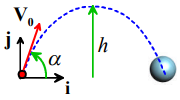If air resistance can be neglected, we can regard the earth and the projectile together as a conservative system. We neglect the change in the earth’s kinetic energy. In addition, since the gravitational force acting on the particle is vertical, the particle’s horizontal component of velocity must be constant.

Calculation:

1. Just after launch, the velocity of the particle is v = V0 cosαi + V0 sinαj

2. The kinetic energy of the particle just after launch is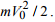Its potential energy is zero.

3. At the peak of the trajectory the vertical velocity is zero. Since the horizontal velocity remains constant, the velocity vector at the peak of the trajectory is v = V0cosαi. The kinetic energy at this point is therefore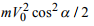4. Energy is conserved, so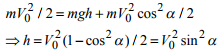Example 2: You are asked to design the packaging for a sensitive instrument. The packaging will be made from an elastic foam, which behaves like a spring. The specifications restrict the maximum acceleration of the instrument to 15g. Estimate the thickness of the packaging that you must use.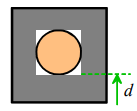This problem can be solved by noting that (i) the max acceleration occurs when the packaging (spring) is fully compressed and so exerts the maximum force on the instrument; (ii) The velocity of the instrument must be zero at this instant, (because the height is a minimum, and the velocity is the derivative of the height); and (iii) The system is conservative, and has zero kinetic energy when the package is dropped, and zero kinetic energy when the spring is fully compressed.

Assumptions:

1. The package is dropped from a height of 1.5m
2. The effects of air resistance during the fall are neglected
3. The foam is idealized as a linear spring, which can be fully compressed.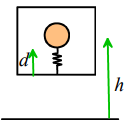Calculations: Let h denote the drop height; let d denote the foam thickness.

1. The potential energy of the system just before the package is dropped is mgh

2. The potential energy of the system at the instant when the foam is compressed to its maximum extent is 1/2 kd2

3. The total energy of the system is constant, so 1/2 kd2 = mgh4. The figure shows a free body diagram for the instrument at the instant of maximum foam compression. The resultant force acting on the instrument is (kd - mg)j, so its acceleration follows as a = (kd / m -g)j . The acceleration must not exceed 15g, so kd / m < 16g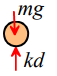5. Dividing (3) by (4) shows that

d > h / 8

The thickness of the protective foam must therefore exceed 18.8cm.

Example 3: The Charpy Impact Test is a way to measure the work of fracture of a material (i.e. the work per unit area required to separate a material into two pieces).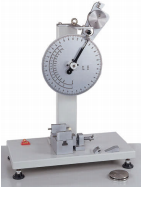It consists of a pendulum, which swings down from a prescribed initial angle to strike a specimen. The pendulum fractures the specimen, and then continues to swing to a new, smaller angle on the other side of the vertical. The scale on the pendulum allows the initial and final angles to be measured. The goal of this example is to deduce a relationship between the angles and the work of fracture of the specimen.

The figure shows the pendulum before and after it hits the specimen.

1. The potential energy of the mass before it is released is V1 = -mgl cosα1 . Its kinetic energy is zero.

2. The potential energy of the mass when it comes to rest after striking the specimen is V2 = -mgl cosα2 . The kinetic energy is again zero.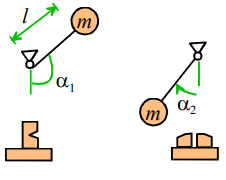The work of fracture is equal to the change in potential energy -

WF = V1 - V2 = mgl (cosα2 - cos α1)

Example 4: Estimate the maximum distance that a long-bow can fire an arrow.

We can do this calculation by idealizing the bow as a spring, and estimating the maximum force that a person could apply to draw the bow. The energy stored in the bow can then be estimated, and energy conservation can be used to estimate the resulting velocity of the arrow.

Assumptions

1. The long-bow will be idealized as a linear spring
2. The maximum draw force is likely to be around 60lbf (270N)
3. The draw length is about 2ft (0.6m)
4. Arrows come with various masses – typical range is between 250-600 grains (16-38 grams)
5. We will neglect the mass of the bow (this is not a very realistic assumption)

Calculation: The calculation needs two steps: (i) we start by calculating the velocity of the arrow just after it is fired. This will be done using the energy conservation law; and (ii) we then calculate the distance traveled by the arrow using the projectile trajectory equations derived in the preceding chapter.

1. Just before the arrow is released, the spring is stretched to its maximum length, and the arrow is stationary. The total energy of the system is T0 + V0 = 1/2 kL2 , where L is the draw length and k is the stiffness of the bow.

2. We can estimate values for the spring stiffness using the draw force: we have that FD = kL, so k = FD / L . Thus T0 + V0 = 1/2 LFD.
3. Just after the arrow is fired, the spring returns to its un-stretched length, and the arrow has velocity V. The total energy of the system is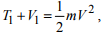, where m is the mass of the arrow
4. The system is conservative, therefore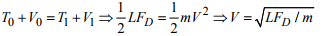5. We suppose that the arrow is launched from the origin at an angle θ to the horizontal. The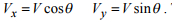The position vector of the arrow can be calculated using the method outlined in Section 3.2.2 – the result is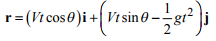We can calculate the distance traveled by noting that its position vector when it lands is di. This gives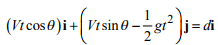where t is the time of flight. The i and j components of this equation can be solved for t and d, with the result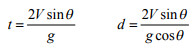The arrow travels furthest when fired at an angle that maximizes sinθ / cos θ - i.e. 45 degrees. The distance follows as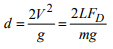6. Substituting numbers gives 2064m for a 250 grain arrow – over a mile! Of course air resistance will reduce this value, and in practice the kinetic energy associated with the motion of the bow and bowstring (neglected here) will reduce the distance.

Example 5: Find a formula for the escape velocity of a space vehicle as a function of altitude above the earths surface.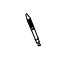The term ‘Escape velocity’ means that the space vehicle has a large enough velocity to completely escape the earth’s gravitational field – i.e. the space vehicle will never stop after being launched.

Assumptions

1. The space vehicle is initially in orbit at an altitude h above the earth’s surface
2. The earth’s radius is 6378.145km
3. While in orbit, a rocket is burned on the vehicle to increase its speed to v (the escape velocity), placing it on a hyperbolic trajectory that will eventually escape the earth’s gravitational field.
4. The Gravitational parameter μ = GM = 3.986012 x 105 km3s-1

(G= gravitational constant; M=mass of earth)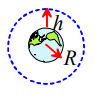Calculation

1. Just after the rocket is burned, the potential energy of the system is V = -GMm / (R + h) , while its kinetic energy its kinetic energy is T = mv2 / 2

2. When it escapes the earth’s gravitational field (at an infinite height above the earth’s surface) the potential energy is zero. At the critical escape velocity, the velocity of the spacecraft at this point drops to zero. The total energy at escape is therefore zero.

3. This is a conservative system, so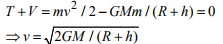4. A typical low earth orbit has altitude of 250km. For this altitude the escape velocity is 10.9km/sec.

Offer running on EduRev: Apply code STAYHOME200 to get INR 200 off on our premium plan EduRev Infinity!

,

,

,

,

,

,

,

,

,

,

,

,

,

,

,

,

,

,

,

,

,

,

,

,

,

,

,

;